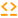# Bound

 Block Group: Number Formatting Icon:The Bound block returns the input number if the input is within the defined minimum and maximum. If the number is above the maximum, this block returns the maximum; if the number is below the minimum, this block returns the minimum.

For information on using dataflow blocks, see Dataflow.

For accepted and excluded arguments of Number Formatting blocks, see Number Formatting.

## Input/Output Properties

The following properties of the Bound block can take input and give output.

• input (number)
• min (number)
• max (number)

input specifies the number to compare to the range.

min specifies the minimum of the range.

max specifies the maximum of the range.

## Output Properties

The following properties of the Bound block can give output but cannot take input.

• inputOutOfRange (boolean)
• output (number)

inputOutOfRange returns TRUE if the input is outside the range, FALSE otherwise.

output returns the input number if it is within the range, the min value if input is below the range, or the max value if input is above the range.

## Examples

The following image shows three examples of the Bound block. In the topmost Bound block, the input is returned. In the middle Bound block, the input is above the range, so the maximum is returned. In the bottommost Bound block, the input is below the range, so the minimum is returned.